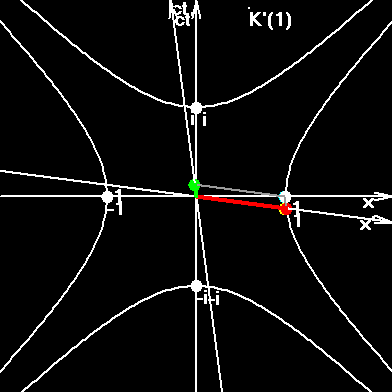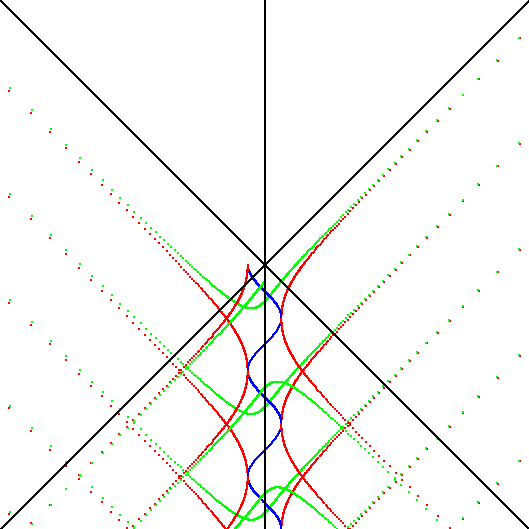This page is slightly obsolete now, but it can help you to understand the Arc Effect. GraviThermal Coil was transformed into rotating Inertio-Thermal Coil, and was renamed as Russian Toroidal Matryoshka, which can be used to perform the press-nuclear reactions with catalyst. The Arc Effect is now proved by several methods. The classic equation of hydrostatic equilibrium is wrong, but it still used in astrophysics now. New equation, containing the Arc Effect item, will ruin the erroneous astrophysics, but we need more time to do it. The science is very conservative. If you want to see the difference in stellar structure while using different equations, open the program Arc Effect (exe-file).

Gravity-Thermal Coil

### Formula of Invention

(Shortened translation from Russian into English)

The pressure of a gas under the constant temperature approximately follows the barometric formula:

p = p0exp(-mgh/(kT)), (1)

where:
p - pressure at the height h;
p0 - pressure at height h = 0;
m - mass of molecule;
g - acceleration at height h;
h - height;
k - Boltzmann constant;
T - temperature.

If we move down the mine, h is negative, pressure grows. If heights/depths much smaller of the Earth's radius, then we can neglect the change of g, which occurs as a result of change of attractive mass. But neglecting the change in g, which occurs as a result of spherical form of the Earth, will lead to large errors. Analysis shows that g, used in the formula above must be changed by gint, here called "internal acceleration". Internal acceleration consists of two parts:

gint = gext - gt = v2ext/r - v2t/r, (2)

where:
vext - the first cosmic velocity at the distance r from the center of the Earth;
vt - average thermal velocity of gas molecule;
gext - Newtonian or external acceleration.
(The mine/tube must have the same temperature as the gas)

From formula 2 we can determine that the higher the temperature of the gas, the smaller the internal acceleration. For an ideal gas with molar mass, equal to that of atomic hydrogen, and at a temperature of about 2500 K, gint becomes equal to zero. As the temperature rises above 2500 K, gint becomes negative. This marks the grows of pressure and density up in the tube.

So, the main sign (indication) of the invention is the usage in device of the more precise barometric formula:

p = p0exp(-mginth/(kT)). (3)

Practical usage of this expression is concluded in the following (see Figure 1):

From container (1) with the help of compressor (2) the working gas fills the isothermal tube at pressure p0 and temperature T1. After achieving equilibrium, the gas in the bottom part of the first vertical tube will have: p1 = p0exp(-mgint1h/kT1), and the same temperature T1. Then gas goes through horizontal isobaric tube to hot tube. At the end of this tube the gas will have the same pressure p1, and high temperature T2. At the top end of the second vertical tube, at equilibrium, the gas will have the pressure:

p2 = p1exp(-mgint2h/(kT2)), (4)

or according to formula 3:

p2 = p0exp(-mh/k*(gint1/T1 - gint2/T2)), (5))

At the bottom of the third tube:

p3 = p0exp(-mh/k*(2gint1/T1 - gint2/T2)). (6))

At the top of the forth tube, or the end of the second spire:

p4 = p0exp(-2mh/k*(gint1/T1 - gint2/T2)). (7))

At the end of i-th spire:

pi = p0exp(-nmh/k*(gint1/T1 - gint2/T2)). (8))

From expression 8, we can conclude that pressure can be risen theoretically unlimitedly.

At the end of the coil the equipment for reactions can be installed. The methods of cooling and heating of the tubes and gas can be different. The main point of this invention is the discovery of dependence of pressure's growth and it's sign from the thermal velocities of gas molecules.

p = p0exp(-mginth/(kT)), gint = gext - gt = v2ext/r - v2t/r. (9)

This equation is new. The convincing evidence for this assertion is the fact that contemporary astrophysics uses an erroneous equation of hydrostatic equilibrium. Then they construct the models of stars and planets:

dp = - rgdr. (10)

The more precise equation, according to this invention will have the form:

dp = - r(gext - gt)dr. (11)

This means, that stars and planets have internal cavities, filled by rarefied gas and photons. The generally accepted astrophysics asserts just the opposite, that stars and planets are densest in their centers. This difference clearly shows the novelty of the invention. Experimental examination of this invention will show us what is at the centers of stars and planets. The last is very important for seismology.

In contemporary thermonuclear devices, where the temperature reaches millions K, it is impossible to neglect the coefficient gint, because at these temperatures, gint is thousands of times greater then Newtonian g and has the opposite sign. This probably just leads to the appearance of convective currents within the plasma and destruction of plasma cord.

Exchanging the entry and exit points to the coil will change the pressure increase into a pressure decrease. This will give us the possibility to build the deep vacuum devices.

(The paper was submitted to the Russian patent agency on the 21 of June 2000, # 2000115803)

To index of Space Genetics

Ivan Gorelik.

My Curriculum Vitae.

My VB-program SR2007.exe proves that the electron is not a point, but a string, embracing the whole Universe in a period, equal to electron's classic period.Particles sew and stitch the space-time, constantly recharging electric and colour field, which are constituent subspaces of our whole macroscopic space-time.Interested? Then go to the index of Space Genetics

Смотрите www.rosokna-tver.ru окна пвх тверь.